First principle investigation of the electronic and thermoelectric properties of Mg2C
Department of Physics, Panjab University, Chandigarh-160014 (India)

† Corresponding author. E-mail: kulwinderphysics@gmail.com

‡ Corresponding author. E-mail: ranjan@pu.ac.in

Kulwinder Kaur thanks Council of Scientific & Industrial Research (CSIR), India for providing fellowship.

Abstract
Abstract

In this paper, electronic and thermoelectric properties of Mg2C are investigated by using first principle pseudo potential method based on density functional theory and Boltzmann transport equations. We calculate the lattice parameters, bulk modulus, band gap and thermoelectric properties (Seebeck coefficient, electrical conductivity, and thermal conductivity) of this material at different temperatures and compare them with available experimental and other theoretical data. The calculations show that Mg2C is indirect band semiconductor with a band gap of 0.75 eV. The negative value of Seebeck coefficient shows that the conduction is due to electrons. The electrical conductivity decreases with temperature and Power factor (PF) increases with temperature. The thermoelectric properties of Mg2C have been calculated in a temperature range of 100 K–1200 K.

1. Introduction

Thermoelectric materials and their applications provide an option of improving the sustainability of electricity which is generated from the waste heat by means of thermoelectric generators that convert heat energy directly into electricity and offer many benefits like moderate efficiency, simple device structure and no moving parts. Thermoelectric-based generators and refrigerators are solid state devices in which heat energy is converted into electricity and vice versa. Thermoelectric generators have been used in niche applications such as power generation or remote power sources. Thermoelectric refrigerator is environment friendly and has long life, and potential to replace traditional air conditioner. Semiconducting alkaline earth metal compounds have been of immense interest for use in thermoelectric device applications.[2,3] One important parameter, which evaluates the performance of thermoelectric material, is a dimensionless figure of merit ZT, which is defined as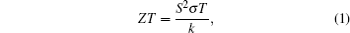where S is the Seebeck coefficient (V/K), σ is the electrical conductivity (S/m), k is the total thermal conductivity (W/mK), and T is the temperature (K). The product of Seebeck coefficient and electrical conductivity (S2σ) is called power factor (PF) and a large power factor means that a device can output large voltage and current. The efficiency (η) of any thermoelectric generator or refrigerator depends on this dimensionless figure of merit ZT.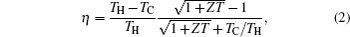where TH and TC are referred to as the temperatures of the hot and cold sides of the sample, respectively.

Good thermoelectric material has high thermo power and low thermal conductivity. High performance thermoelectric material is usually considered to have ZT equal to or greater than one. Magnesium compounds Mg2X (X = Si, Ge, Sn) and their solid solutions are of great interest as promising thermoelectric materials in a temperature range from 500 K to 900 K. These materials are semiconductors having antifluorite structure. The antifluorite semiconductor is important because it forms metal-semiconductor hybrid material. Mg2Si and Mg2Ge are good materials for technological applications as infrared detectors in a wavelength range of 1.2 μm–1.8 μm for optical fiber. Carbides, which have been intensively studied for more than half a century, still remain a major center of scientific and technological attention. Magnesium compounds containing Mg–C and C–C bonds are quite fascinating from both fundamental science and synthesis perspectives.[9,10] The carbon has two Mg–C bonds and a lone pair. The two magnesium atoms are bonded by single Mg–Mg bond. The carbon center is much like an organic carbene. The nature of chemical bond presents and determines the properties of Mg2C. There is no covalent C–C bond in Mg2C. So these types of materials are ionic semiconductors. sp3 and sp2 carbon network are intercalated with Mg[12,13] and novel polymeric carbide. The nature of Mg–C chemical bond has great importance in polar organometallic compounds and in the covalent /ionic nature of carbanions.[14,15] There are four magnesium carbides, which are well known as (i) tetragonal MgC2, (ii) orthorhombic α-Mg2C3, (iii) monoclinic β-Mg2C3, and (iv) cubic Mg2C. In the present paper, we will discuss the electronic and thermoelectric properties of cubic Mg2C because this phase exists at high temperature and pressure. It was theoretically investigated by Cork ill and Cohen in 1993. And recently synthesized by Kurakevych et al. at high pressure conditions, this compound forms from the elements at pressure above 15 GPa and stable in ambient Fm3m conditions. Owing to small size of C atom, it has large elastic modulus, which is related to hardness. This material is good for high temperature and pressure applications. This compound is unique in the sense that carbon is stabilized in a rare C4– ionic state, which is extremely rare for alkaline–earth metal carbides. Carbon is eight fold coordinated by magnesium, and magnesium has four fold coordinated with carbon. This compound is easily hydrolyzed by the moisture in air, so it must be handled carefully. Li et al. have investigated the electronic and lattice vibrational properties of Mg2C by the pseudo potential method. They found that this compound is small gap semiconductor. Laref et al. reported the mechanical, electronic and optical properties of this compound. Recent study by Chernatynskiy and Phillpot revealed that the calculated thermal conductivity is 34 W/mK for Mg2C at 300 °C. Theoretical and experimental studies of thermoelectric properties for Mg2C system are not yet available in the literature. These calculations may serve as the guidance for researchers in future. The aim of the present work is to investigate the electronic properties by using density functional theory and thermoelectric properties by using phonon Boltzmann transport equation (pBTE) and Boltzmann transport equations.

2. Theoretical and computational approach

Mg2C is face centered cubic (FCC) structures with primitive translation vectors a = a(0,1/2,1/2), b = a(1/2,0,1/2), and c = a(1/2,1/2,0) where a is lattice parameter. The structure symmetry of this compound is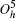, the corresponding space group is Fm-3m (group No. 225). Three in-equivalent sites can be specified in the irreducible unit cell, namely C : a(0,0,0), Mg : a(1/4,1/4,1/4), and Mg : a(1/4,1/4,3/4). This compound belongs to the antifluorite structure family and semiconductor in nature. We considered super cell 2 × 1 × 1 of Mg2C conventional unit cell which contains 24 atoms (16 Mg atoms and 8 C atoms).

The present calculations were performed using density functional theory (DFT) with the Perdew–Burke–Ernzerhof (PBE) generalized gradient approximation (GGA) and using plane wave pseudo potential method as implemented in Quantum Espresso package. Norm-conserving pseudo potential based on the Troullier–Martins scheme were utilized to model core electrons. The cutoff for the kinetic energy was set to be 70 Ry (1 Ry = 13.6056923 eV) for the plane-wave expansion of the electronic wave function. The marzari-Vanderbilt smearing size was fixed to be 0.003 Ry. The internal lattice pressure was smaller than 0.2 kbar (1 bar = 105 Pa). The Brillouin zone integration was performed over a Monkhorst–Pack 5 × 10 × 10 meshes. The lattice constant of Mg2C was optimized until the total energy converged to at least 10−8 Ry, the forces between atoms became smaller than 10−5 Ry/bohr. A denser k-mesh of 16 × 16 × 16 was used for calculating the density of states. A very fine mesh of 58 × 58 × 58 points was used to determine the final electronic structure for use in the electronic thermoelectric properties calculations. BoltzTraP code was used to find the electronic thermoelectric properties (Seebeck coefficient (S), electrical conductivity (σ), and electronic thermal conductivity (ke), which is based on constant time approximation and rigid band approximation [RBA].[25,26] To calculate transport properties, the following Boltzmann semi classic equations are used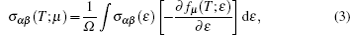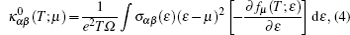where k0 is the electronic part of the thermal conductivity. The Seebeck coefficient can be easily calculated as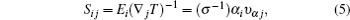where υαj is the band velocity, μ is the chemical potential, ɛ is band energy, and fμ is the Fermi function.

In RBA approximation the band structure remains unchanged as the Fermi level is moved up and down. Shifting the Fermi level only results in changes of electron and hole concentrations because the donor and acceptor doping levels are varied. With this code, the thermoelectric properties were calculated with respect to the relaxation time (τ). To calculate lattice thermal conductivity (kl), ShengBTE code based on the phonon Boltzmann transport equation (pBTE) was used. This code is based on the second-order (harmonic) and third-order (an harmonic) interatomic force constants (IFCs) combined with a full solution of the pBTE and successfully predicts the lattice thermal conductivity (kl).[28,29] The lattice parameter and bulk modulus were calculated by computing the total energy for different volumes and fitted to Murnaghan’s equation of state: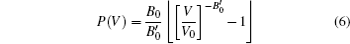where P is the pressure, V0 is the reference volume, V is the deformed volume, B0 is the bulk modulus, and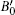is the derivative of the bulk modulus with respect to pressure.

3. Results and discussion
3.1. Electronic properties

Our calculated lattice constant of Mg2C is 5.443 Å, which is in better agreement with experimental data reported by Kurakevych et al. as compared with the previously reported theoretical data by Kalarasse and Bennecer. Comparisons among other reported theoretical and experimental data of calculated lattice constant, bulk modulus, band gap have shown in Table 1.Table 1.

Lattice constants, band gaps and bulk moduli of Mg2C.

.

Figure 1 shows the indirect band structure of Mg2C. In this structure the valence band maximum (VBM) lies at Γ point and conduction band minimum lies at X point. The valence band is split into two sub bands. The lower valence band is due to the contributions from C s states and Mg s states. The upper valence band near the Fermi level is due to C atom p states and has a band gap of 0.7524 eV. The calculated gap is in good agreement with previously data reported by Kalarasse and Bennecer as shown in Table 1. The lower valance band near −5 eV is mainly C atom s states and small contribution of Mg atom s states and upper valance band near 0 eV to 5 eV is due to C atom p states and Mg atom s, p states. The number of electrons within the Fermi surface can be used to find the nature of material. To have an explicit understanding of interaction between the different orbitals of atoms, total and projected density of states are calculated. Figures 2 and 3 present the detailed calculations of total and partial density of states, where the contribution of each atomic orbitals for each element is shown. The lowest valance band originates from s-states of C atom and s state of Mg atom. The highest band is due to the s and p hybridizations, which originate from Mg cation. The main contribution to the antibonding state comes from p orbital of the anion C and in lowest magnitude, from the p orbital of Mg. The conduction band is the mixture of Mg p and s states with C p and s states.

 Figure Option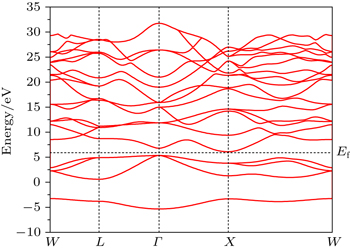Fig. 1. Band structure of Mg2C.
 Figure Option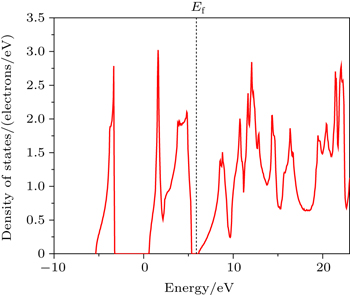Fig. 2. Density of states (DOS).
 Figure Option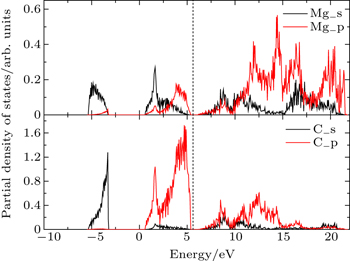Fig. 3. Projected density of states (PDOS).
3.2. Thermoelectric properties

In this Subsection, thermoelectric properties are presented. Figure 4 shows the variation of Seebeck coefficient (S) with temperature. The sign of calculated Seebeck coefficient is negative, which indicates that the conduction is due to electrons. The absolute value of Seebeck coefficient increases with temperature. The relation of Seebeck coefficient with carrier concentration is given below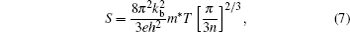where kb is the Boltzmann constant, m* is the effective mass, and n is the carrier concentration. According to Eq. (8), Seebeck coefficient is inversely proportional to carrier concentration. Increase in Seebeck coefficient with temperature increasing is due to low concentration of charge carriers.

 Figure Option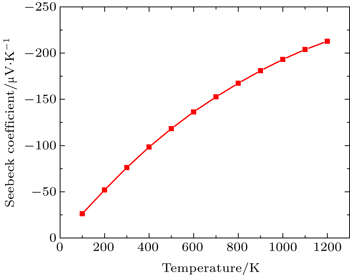Fig. 4. Variation of Seebeck coefficient (S) with temperature.

Figure 5 shows the variation of electrical conductivity with temperature. Electrical conductivity is directly proportional to carrier concentration (n) as given by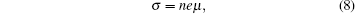where n is carrier concentration and μ is the carrier mobility. In the present work the electrical conductivity (σ/τ) decreases with temperature increasing, which is due to the change of mobility. LaBotz et al. and Noda et al. revealed that the mobility depends on the temperature with the relation (μT−3/2) in Mg2SixGe1−x, where acoustic lattice scattering is predominant mechanism. Electrical conductivity depends on not only the carrier concentrations, but also mobility. Therefore, electrical conductivity decreases due to mobility decreasing with temperature.

 Figure Option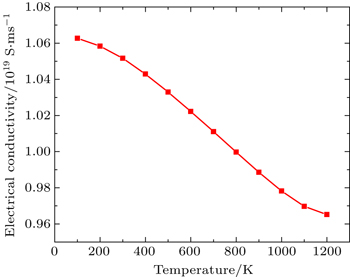Fig. 5. Variation of electrical conductivity (σ τ) with temperature.

Total thermal conductivity is sum of electronic (ke) and lattice part (kl) of crystal as given by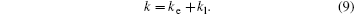Figure 6 shows the variation of electronic contribution of thermal conductivity with temperature. ke increases with temperature increasing. With rise in temperature, the charge carriers are thermally excited, thus increasing the electronic thermal conductivity. The lattice contribution of thermal conductivity also decreases with increasing temperature (Fig. 7), which indicates the increase in phonon scattering. The lattice thermal conductivity is inversely proportional to temperature which indicates that the phonon–phonon interaction is a main source of the lattice thermal resistance. We find that 99% thermal conductivity is due to lattice contribution. The lattice contribution plays a bigger role than electronic contribution. The total thermal conductivity is dominated by lattice contribution.

 Figure Option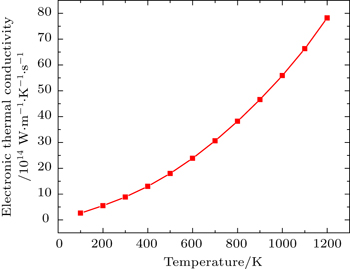Fig. 6. Electronic thermal conductivity (ke/τ) versus temperature.
 Figure Option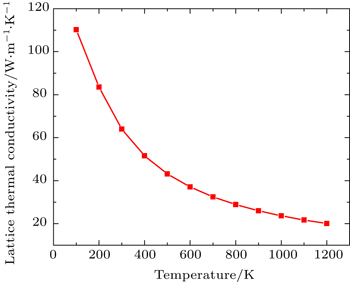Fig. 7. Lattice thermal conductivity (kl) versus temperature.

Figure 8 shows the variation of power factor with temperature. With the increase of temperature the power factor increases. The lattice thermal conductivity plays a key role in thermoelectric material. But in this compound the thermal conductivity is very high due to phonon–phonon interactions. The figure of merit (ZT) is very low for Mg2C due to high value of thermal conductivity.

 Figure Option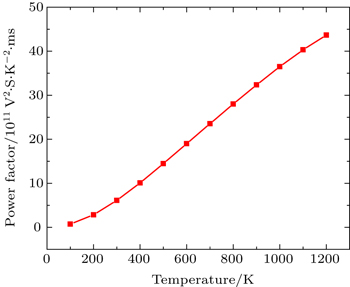Fig. 8. Power factor (S2σ/τ) versus temperature.
4. Conclusions

By using first principle calculations, the electronic and thermoelectric properties of pure Mg2C are investigated. The calculated result shows that Mg2C exhibits the n-type conduction, i.e., the conduction is due to electrons, and also it is confirmed that Mg2C is an indirect semiconductor. Thermoelectric studies show that Seebeck coefficient increases with increasing temperature. The electrical conductivity decreases with increasing temperature due to change of mobility. The electronic part of thermal conductivity (ke) increases with increasing temperature and lattice contribution decreases with increasing temperature. The calculated result proves that the figure of merit (ZT) is very low.

Reference
 1 Wood C1988Rep. Prog. Phys.51459 2 Liu ZWatanabe MHanabusa M 2001 Thin Solid Films 381 262 3 Borisenko E VFilonov A B2000General Material AspectsBerlinSpringer-Verlag179 4 DiSalvo F J 1999 Science 285 703 5 Snyder G JUrsell T S 2003 Phys. Rev. Lett. 91 148301 6 Morris R GRedin R DDanielson G C 1958 Phys. Rev. 109 1909 7 Akasaka MIida TMatsumoto AYamanaka KTakanashi YImai THamada N 2008 J. Appl. Phys. 104 13703 8 Kurakevych O O 2009 J. Superhard Mater. 31 139 9 Lee C HLambrecht W R LSegall B 1995 Phys. Rev. B 51 10392 10 Srepusharawoot PBlomqvist AAraujo C MScheicher R HAhuja R 2010 Phys. Rev. B 82 125439 11 Corkill J LCohen M L 1993 Phys. Rev. B 48 17138 12 Karttunen JFassler T FLinnolahti MPakkanen T A 2011 Inorg. Chem. 50 1733 13 Yamanaka S 2010 Dalton Trans. 39 1901 14 Lambert CSchleyer P V R 1994 Angew. Chem. 106 1187 15 Bickelhaupt F Mvan Eikema Hommes N J RFonseca Guerra CBaerends E J 1996 Organometallics 15 2923 16 Kurakevych O OStrobel T AKim D YCody G D 2013 Angew. Chem. Int. Ed. 52 8930 17 Li TJu WLiu HCui HZhao XYong YFeng Z 2014 Comput. Mater. Sci. 93 234 18 Laref SLaref A 2008 Comput. Matt. Sci. 44 664 19 Chernatynskiy APhillpot S R 2015 Phys. Rev. B 92 064303 20 Ziman J M2001Electrons and Phonons: The Theory of Transport Phenomena in SolidsOxford Classics Series, OxfordClarendon Press 21 Perdew J PBurke KErnzerhof M1996Phys. Rev. Lett.773864 22 Giannozzi PBaroni SBonini Net al. 2009 J. Phys.: Condens. Matter 21 395502 23 Monkhorst H JPack J D 1976 Phys. Rev. B 13 5188 24 Madsen G K HSingh D J 2006 Comput. Phys. Commun. 175 67 25 Scheidemantel T JAmbrosch-Draxl CThonhauser TBadding J VSofo J O 2003 Phys. Rev. B 68 125210 26 Jodin LTobola JPecheur PScherrer HKaprzyk S 2004 Phys. Rev. B 70 184207 27 Li WCarrete JKatcho N AMingo N 2014 Comput. Phys. Commun. 185 1747 28 Ma JLi WLuo X 2014 Appl. Phys. Lett. 105 082103 29 Carrete JMingo NCurtarolo S 2014 Appl. Phys. Lett. 105 101907 30 Murnaghan F D 1944 Proc Natl. Acad. Sci. USA 30 244 31 Kalarasse FBennecer B 2008 J. Phys. Chem. Solids 69 1775 32 Kurakevych O OGodec Y L 2014 J. Phys. Chem. C 118 8128 33 LaBotz R JMason D RO’Kane D F 1963 J. Electrochem. Soc. 110 127 34 Noda YKon HFurukawa YOtsuka NNishida I AMasumoto K 1992 Mater. Trans. JIM 33 851